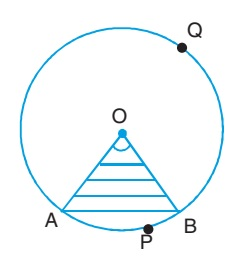# Perimeter and Area of Sector

A part of a circular region enclosed between two radii of the corresponding circle is called a sector of the circle.

The shaded region OAPB is a sector of the circle with centre O. ∠AOB is called the central angle or simply the angle of the sector.APB is the corresponding arc of this sector. The part OAQB (unshaded region) is also a sector of this circle. OAPB is called the minor sector and OAQB is called the major sector of the circle (with major arc AQB).

### Perimeter of Sector

The perimeter of the sector OAPB is equal to OA + OB + length of arc APB.

Let radius OA (or OB) be r, length of the arc APB be l and ∠AOB be θ.

You can find the length l of the arc APB as follows:

Circumference of the circle = 2πr

Now, for total angle 360° at the centre, length = 2πr

So, for angle θ,

$$l = \frac{2 \pi r}{360} \times \theta$$

### Area of Sector

Area of the circle = πr2

Now, for total angle 360°, area = πr2

So, for angle θ,

$$\text{area} = \frac{\pi r^2}{360} \times \theta$$

Example 1: Find the perimeter and area of the sector of a circle of radius 6 cm and length of the arc of the sector as 22 cm.

Perimeter of the sector = 2r + length of the arc

= (2 × 6 + 22) cm = 34 cm

For area, first find the central angle θ.

πrθ/180° = 22

θ = 210°

So, area of the sector = πr2θ/360°

= 66 cm2